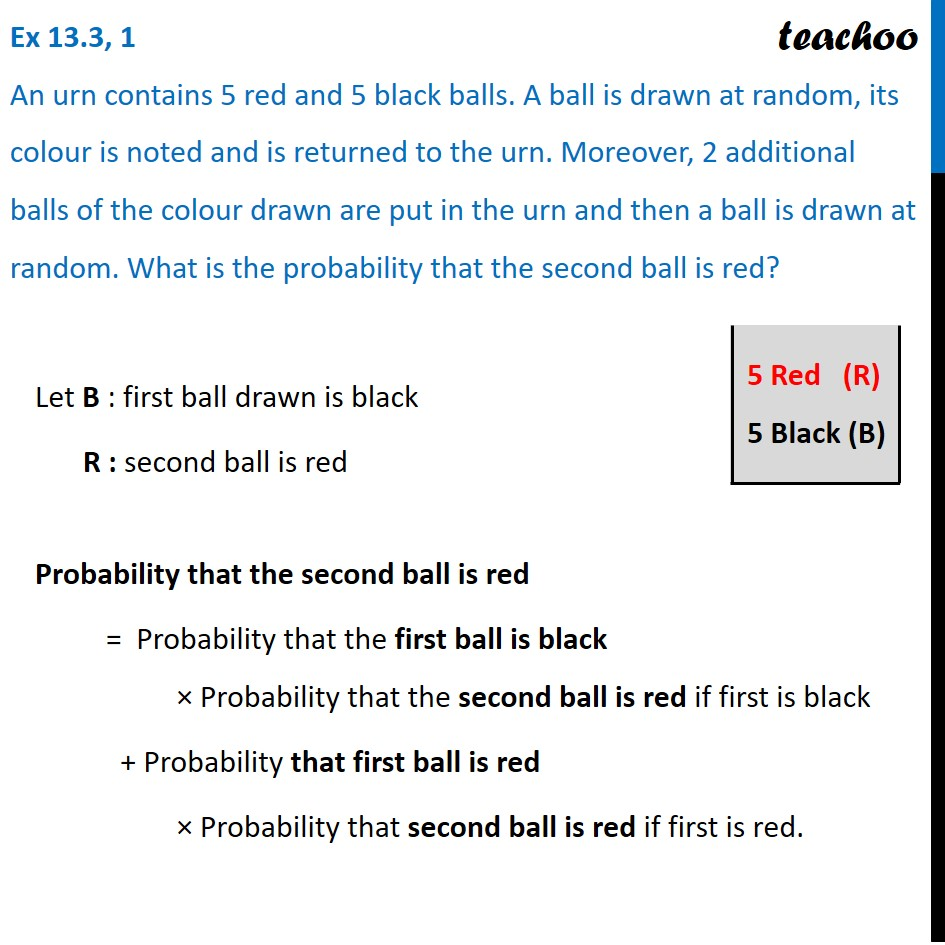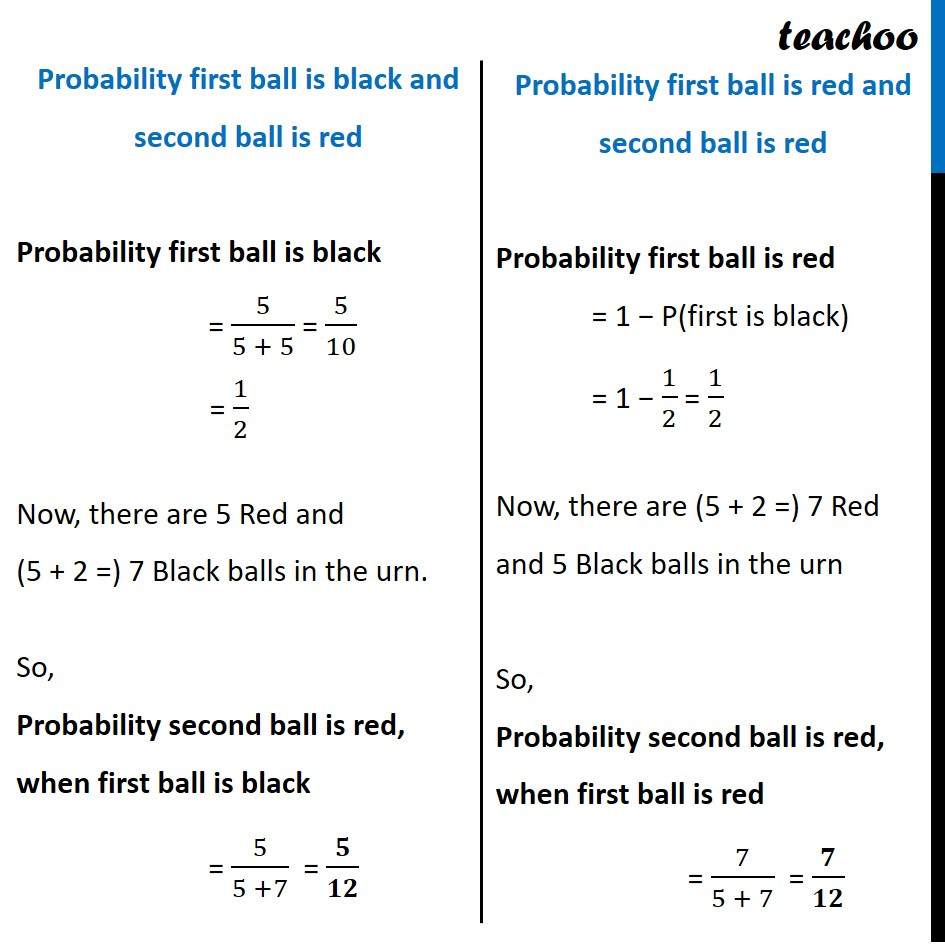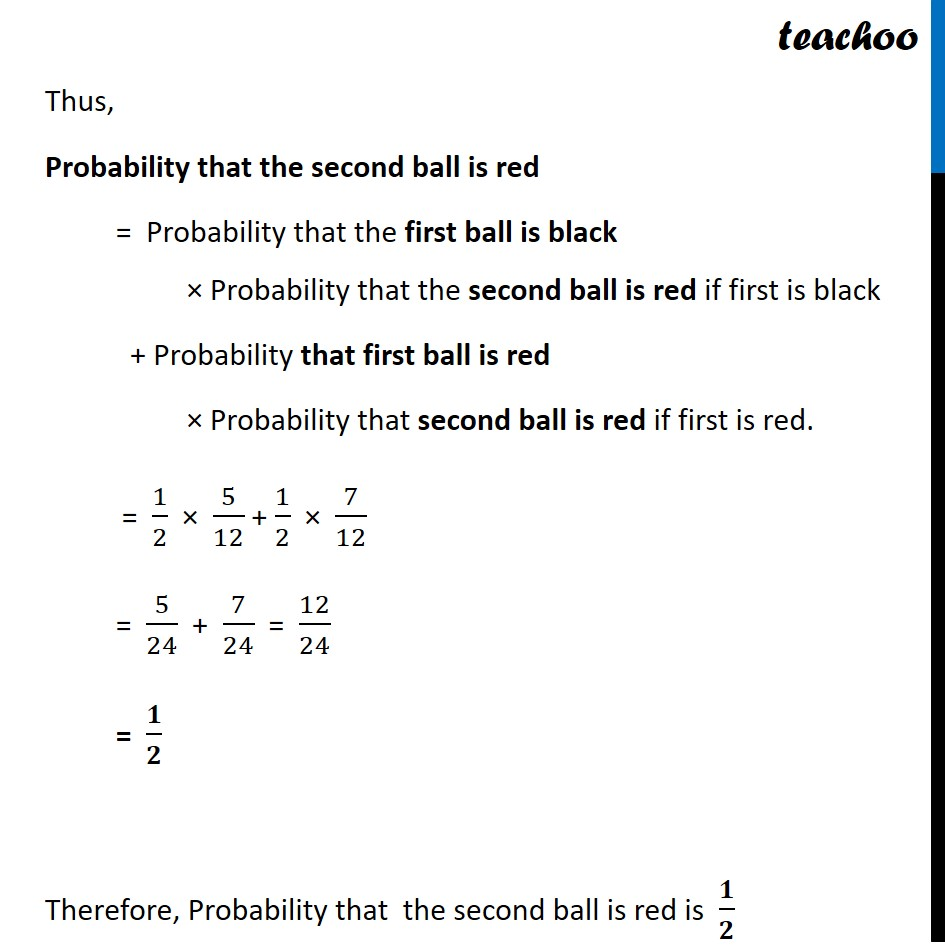Ex 13.3

Chapter 13 Class 12 Probability
Serial order wiseLearn in your speed, with individual attention - Teachoo Maths 1-on-1 Class

### Transcript

Ex 13.3, 1 An urn contains 5 red and 5 black balls. A ball is drawn at random, its colour is noted and is returned to the urn. Moreover, 2 additional balls of the colour drawn are put in the urn and then a ball is drawn at random. What is the probability that the second ball is red?Let B : first ball drawn is black R : second ball is red Probability that the second ball is red = Probability that the first ball is black × Probability that the second ball is red if first is black + Probability that first ball is red × Probability that second ball is red if first is red. Probability first ball is black and second ball is red Probability first ball is black = 5/(5 + 5) = 5/10 = 1/2 Now, there are 5 Red and (5 + 2 =) 7 Black balls in the urn. So, Probability second ball is red, when first ball is black = 5/(5 +7) = 𝟓/𝟏𝟐 Probability first ball is red and second ball is red Probability first ball is red = 1 − P(first is black) = 1 − 1/2 = 1/2 Now, there are (5 + 2 =) 7 Red and 5 Black balls in the urn So, Probability second ball is red, when first ball is red = 7/(5 + 7) = 𝟕/𝟏𝟐 Thus, Probability that the second ball is red = Probability that the first ball is black × Probability that the second ball is red if first is black + Probability that first ball is red × Probability that second ball is red if first is red. = 1/2 × 5/12 + 1/2 × 7/12 = 5/24 + 7/24 = 12/24 = 𝟏/𝟐 Therefore, Probability that the second ball is red is 𝟏/𝟐